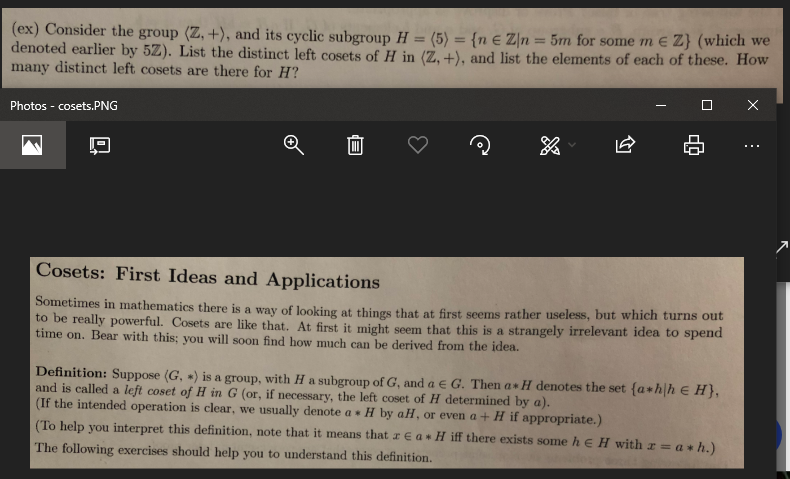(ex) Consider the group (Z, +), and its cyclic subgroup H - (5) (n e Zn 5m for some m edenoted earlier by 52). List the distinct left cosets of H in (Z, +), and list the elements of each of these. Howmany distinct left cosets are there for H?Photos -cosets.PNGCosets: First Ideas and Applicationsin mathematics there is a way of looking at things that at first seems rather useless, but which turns outto bereally powerful. Cosets are like that. At first it might seem that this is a strangely irrelevant idea to spendtime on. Bear with this: you will soon find how much can be derived from the idea.Definition: Suppose (G, ,) is a group, with H a subgroup of G, and a e G. Then a * I denotes the set fa hlh e H),and is called a left coset of H in G (or, if necessary, the left coset of H determined by a)(If the intended operation is clear, we usually denote a H by aH, or even a+ H if appropriate.)To help you interpret this definition, note that it means that z e a * H iff there exists some h e H with zThe following exercises should help you to understand this definitiona * h.

Question

Abstract Algebra

I need help on a problem that requires the definition of Cosets.

The definition is given in the given picture as well as the problem above it.help_outlineImage Transcriptionclose(ex) Consider the group (Z, +), and its cyclic subgroup H - (5) (n e Zn 5m for some m e denoted earlier by 52). List the distinct left cosets of H in (Z, +), and list the elements of each of these. How many distinct left cosets are there for H? Photos -cosets.PNG Cosets: First Ideas and Applications in mathematics there is a way of looking at things that at first seems rather useless, but which turns out to be really powerful. Cosets are like that. At first it might seem that this is a strangely irrelevant idea to spend time on. Bear with this: you will soon find how much can be derived from the idea. Definition: Suppose (G, ,) is a group, with H a subgroup of G, and a e G. Then a * I denotes the set fa hlh e H), and is called a left coset of H in G (or, if necessary, the left coset of H determined by a) (If the intended operation is clear, we usually denote a H by aH, or even a+ H if appropriate.) To help you interpret this definition, note that it means that z e a * H iff there exists some h e H with z The following exercises should help you to understand this definition a * h. fullscreen
Step 1

Given H = <5> = {n∈ Z | n = 5m, for some integer m}.

The left cosets will be:

0 + H, 1 + H, 2 + H, 3 + H, 4 + H,... and in general a + H, where a is any integer.

Step 2

To find the distinct left cosets.

Step 3

To list the 5 distinc...

Want to see the full answer?

See Solution

Want to see this answer and more?

Our solutions are written by experts, many with advanced degrees, and available 24/7

See Solution
Tagged in

Math